# Quiz question 1 quiz question 2 quiz question

• Slides: 15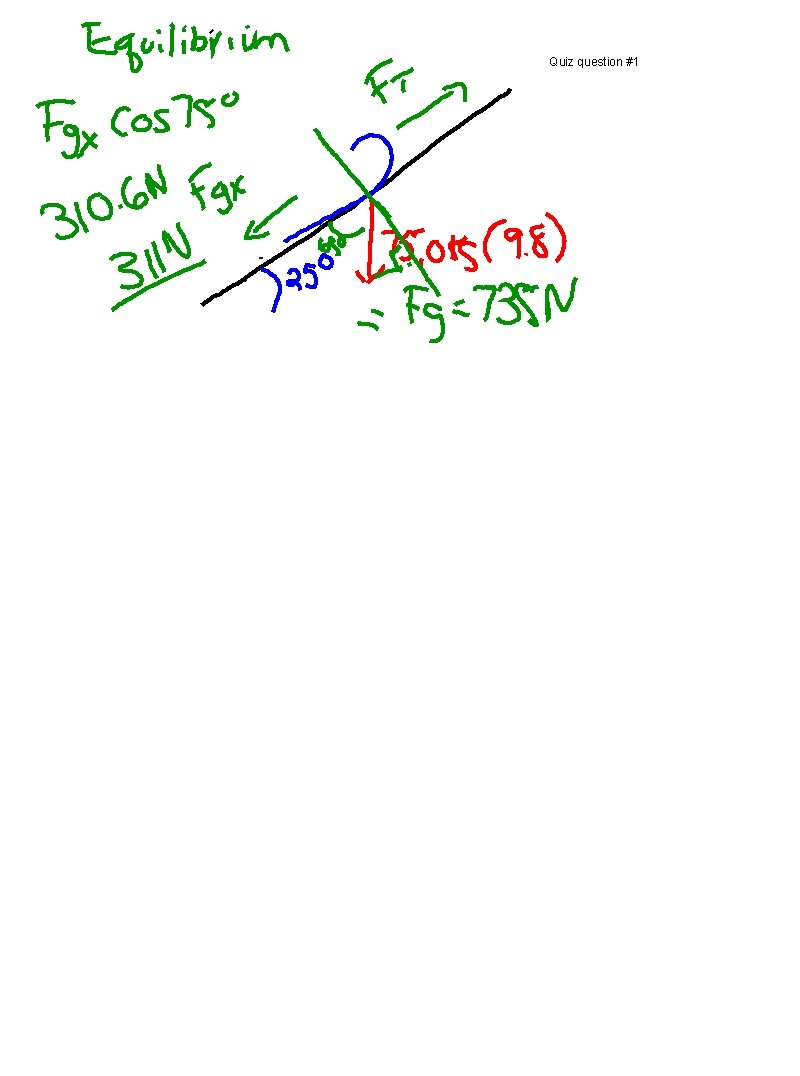Quiz question #1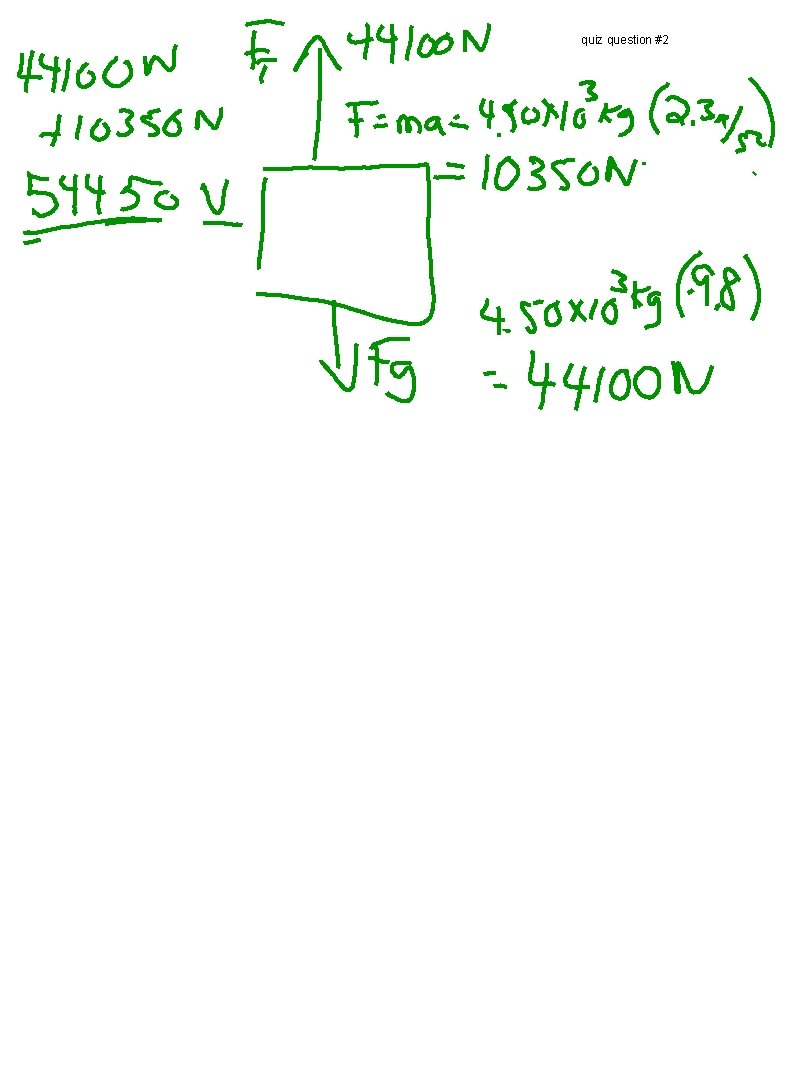quiz question #2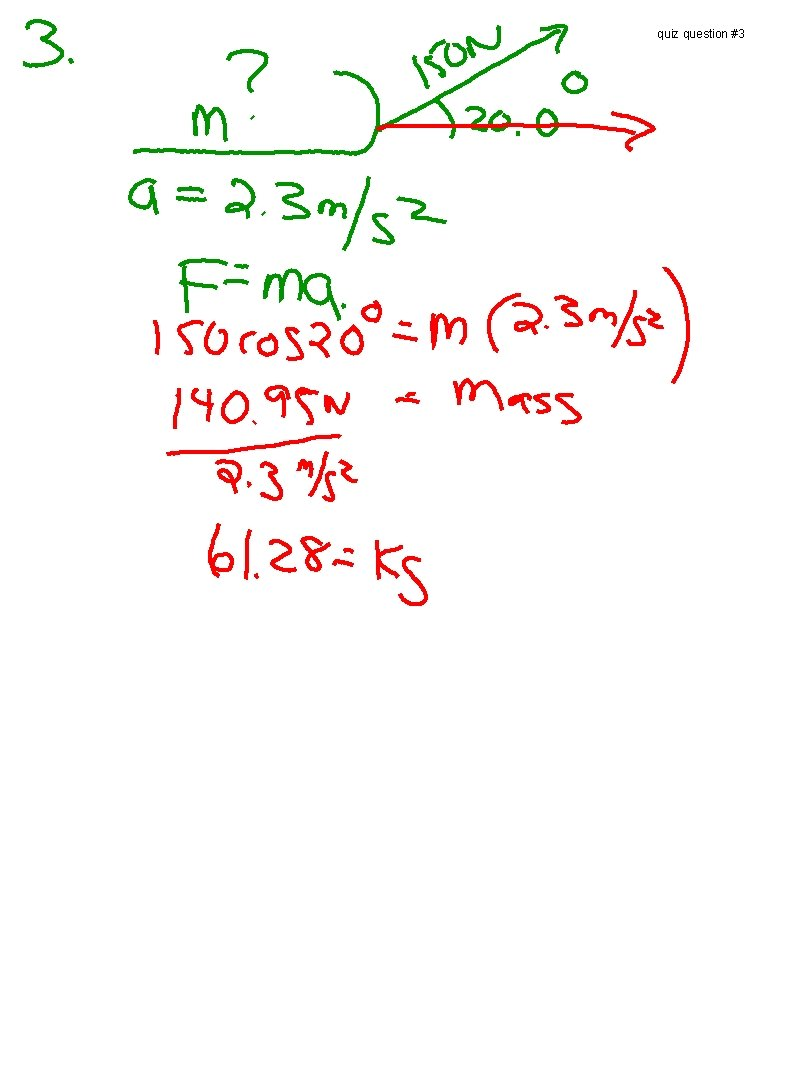quiz question #3Handout question #3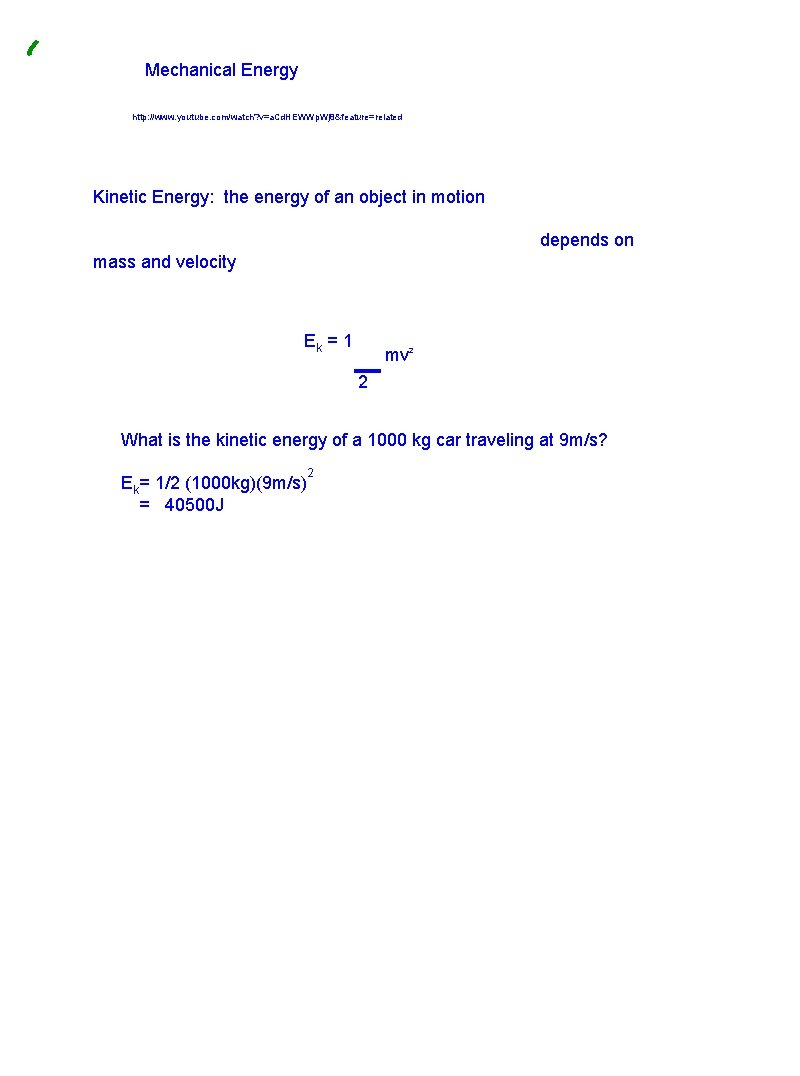Mechanical Energy http: //www. youtube. com/watch? v=a. Cd. HEWWp. Wj 8&feature=related Kinetic Energy: the energy of an object in motion depends on mass and velocity Ek = 1 mv 2 2 What is the kinetic energy of a 1000 kg car traveling at 9 m/s? Ek= 1/2 (1000 kg)(9 m/s) = 40500 J 2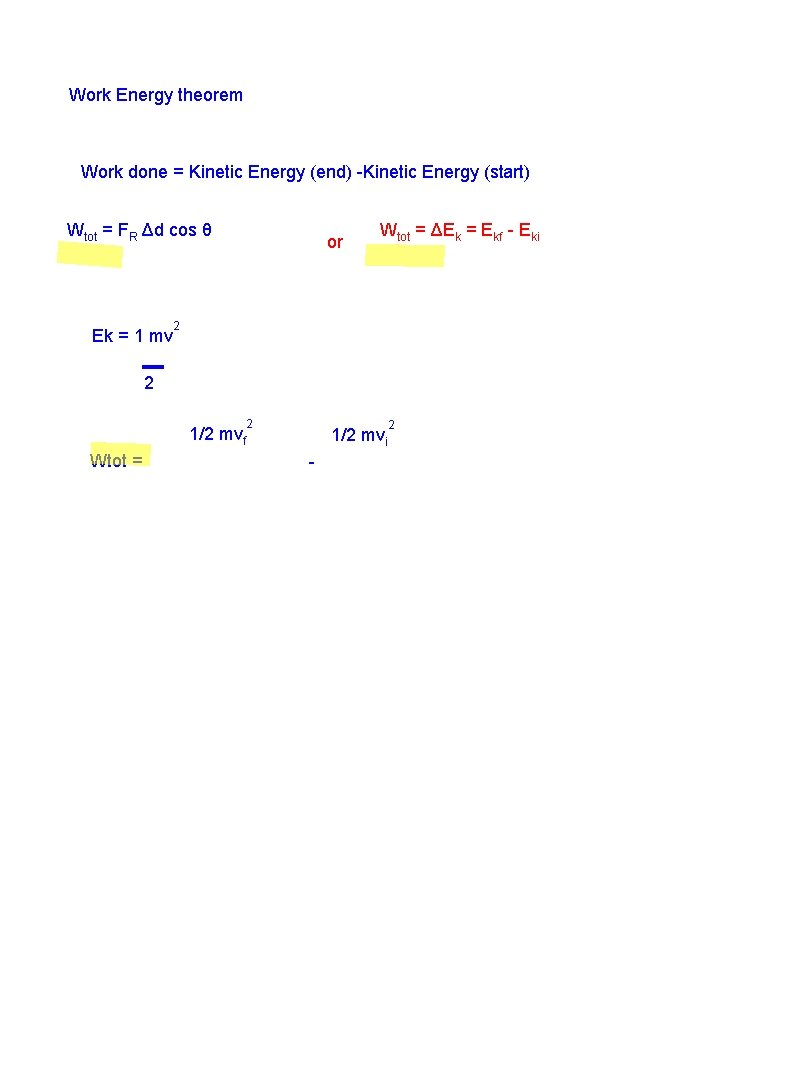Work Energy theorem Work done = Kinetic Energy (end) -Kinetic Energy (start) Wtot = FR Δd cos θ Ek = 1 mv or Wtot = ΔEk = Ekf - Eki 2 2 1/2 Wtot = 2 mvf 2 1/2 mvi -A car is travelling at 15 m/s, it weighs 600 kg What is the kinetic energy? Ek = 1 mv 2 2 Ek = 0. 5(600 kg)(15 m/s) 2 = 67500 Jp. 344 Example B A dog is pulling a 25. 0 kg sled over a horizontal surface of 4. 00 m with a force of 50. 0 N using a horizontal rope. The sled was initially at rest and we assume that friction is negligible. a) What is the total work done? b) What is the sled's final velocity? Data: m= 25. 0 kg F = 50. 0 N 0 θ=0 V i=0 Δd = 4. 0 m Wtot = ? Vf = ? a) W tot = WFT + WFg + WFN Wtot = 50. 0 N 4. 0 m = 200 J b) Eki = 0 (vi = 0) W tot = Ekf 2 200 J = 1/2 m(vf) 2 4 00 J/25. 0 kg= vf v f = 4. 00 m/s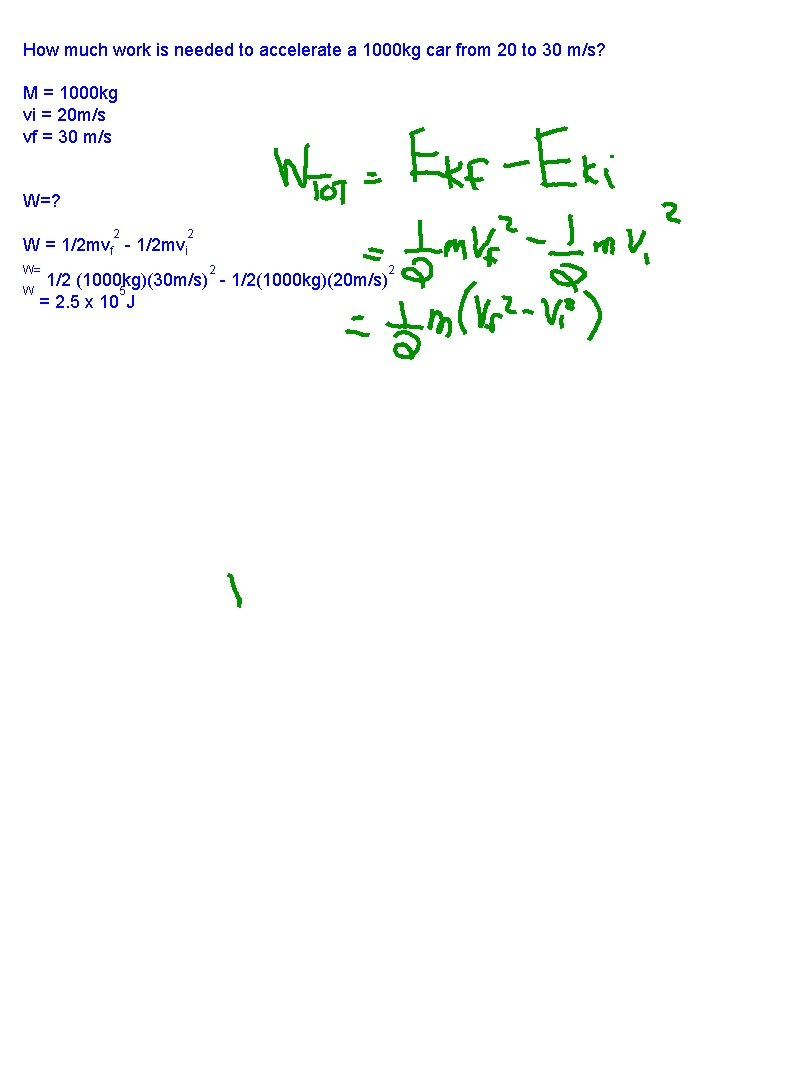How much work is needed to accelerate a 1000 kg car from 20 to 30 m/s? M = 1000 kg vi = 20 m/s vf = 30 m/s W=? 2 2 W = 1/2 mvf - 1/2 mvi W= 2 1/2 (1000 kg)(30 m/s) - 1/2(1000 kg)(20 m/s) W 5 = 2. 5 x 10 J 2Gravitational potential energy Epg = mgh m =. 25 kg Epg - Gravitational potential energy (J) m mass of object 2 h= 2. 0 m g gravitational acceleration (m/s ) h is height above the reference level (usually the ground) expressed in metres (m) 2 Epg = mgh =. 25 kg(9. 8 m/s )(2. 0 m)= 4. 9 JConservation of mechanical energy Mechanical Energy = Kinetic Energy + Potential Energy Em = Ek + Ep Eki + Epi = Ekf + Epf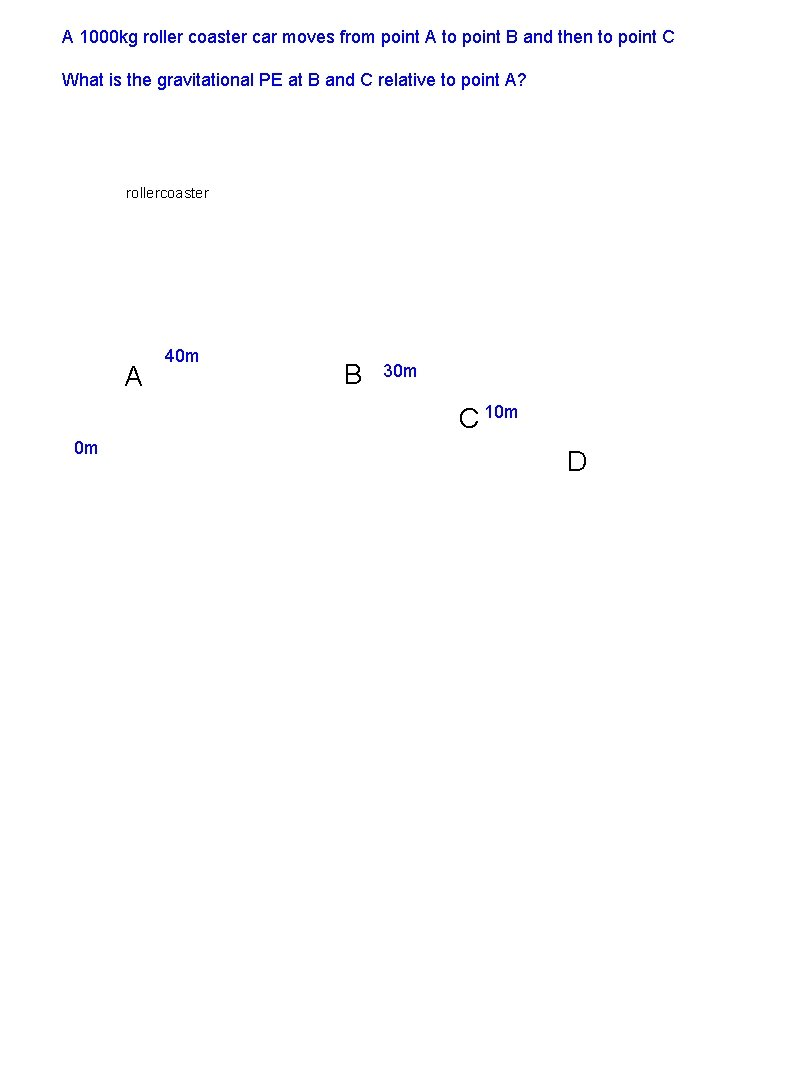A 1000 kg roller coaster car moves from point A to point B and then to point C What is the gravitational PE at B and C relative to point A? rollercoaster A 40 m B 30 m C 10 m 0 m DThe law of conservation of total energy states that the total quantity fo energy is constant in an isolated system. If there is friction part of the mechanical energy is transformed into thermal energy. ∴ Eki + Epi = Ekf + Epf + ∆Eth = the final thermal energy or heat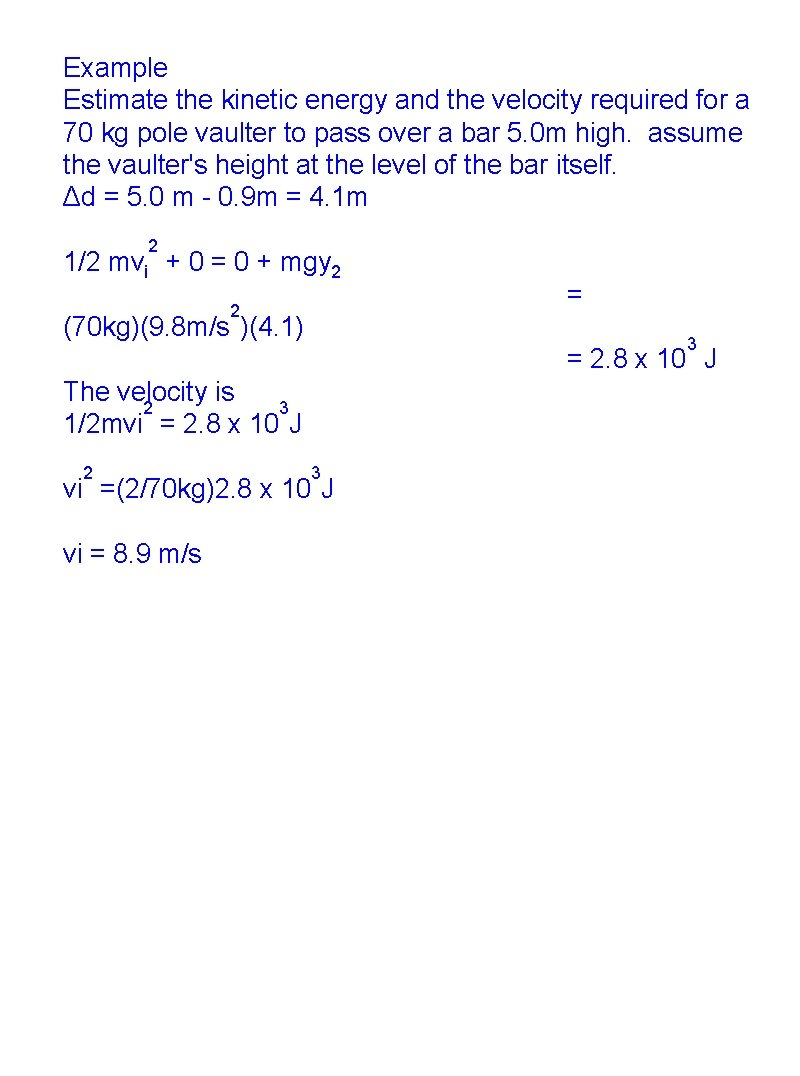Example Estimate the kinetic energy and the velocity required for a 70 kg pole vaulter to pass over a bar 5. 0 m high. assume the vaulter's height at the level of the bar itself. Δd = 5. 0 m - 0. 9 m = 4. 1 m 2 1/2 mvi + 0 = 0 + mgy 2 2 (70 kg)(9. 8 m/s )(4. 1) = 3 = 2. 8 x 10 J The velocity is 2 3 1/2 mvi = 2. 8 x 10 J 2 3 vi =(2/70 kg)2. 8 x 10 J vi = 8. 9 m/s